Introduction and In-depth Explanation To Equations Of Motion With Formula Graph ExamplesIntroduction and In-depth Explanation To Equations Of Motion With Formula Graph Examples

Cricket fan? Soccer fan? Hockey fan? What is the primary thing taught when you begin training for these or other similar sports? That's exactly what motion and speed acceleration are all about. That’s what we call equations of Motion.

Let’s begin with an Introduction to equations of motion for easy comprehension.

Introduction To Equations Of Motion

Equation of Motion is a mathematical formula that defines a person’s velocity, position, and acceleration or position in relation to a particular area of reference. Newton's second law declares that the force F exerted on an object can be calculated as the sum of the body's mass. The speed of its centre of mass F = ma is the most fundamental equation of the Motion of a body in classical mechanics.

Suppose the force acting on a body can be described in relation to time.

1. In that case, the speed and location of the body in terms of time may theoretically be calculated using Newton's equation through a process called integration.

2. For instance, the body that falls accelerates at a constant rate that is g. The term "acceleration" refers to the rate at which the speed of the change in velocity is relative to time.

3. So, when you integrate the velocity v as a function of time, t is calculated by the formula v = gt. Velocity is the speed of changing position S.

4. As a result, when you integrate the velocity equation results in S + 1/2gt2. That is simple!

5. If the force exerted on a body is defined by its velocity or position, then the equation of Newton could be more daunting.

6. If a body is compelled to travel in a predetermined method on a set route, it might be possible to calculate the equation of position and time.

The velocity-time equation and acceleration-time equations may theoretically be obtained using a method known as differentiation.

What are the Three Equations of Motion?

Suppose we consider a motion with continuous or uniform acceleration (one with equal changes in velocity over a similar period in time). We can derive three common equations of Motion, also referred to as the rules of steady acceleration.

These equations consist of displacement(s) and speed ( final and initial), time(t) and acceleration(a) that determine the movement of particles. These equations are only used when the acceleration of a body is constant and the Motion is straight. Here are the three equations,

First Equation: v = u + at

Second Equation: v² = u² + 2as

Third Equation: s = ut + ½at²

where,

S means: displacement

U means: initial velocity;

V means: final velocity

A means: acceleration

T means: time of motion.

First Equation of Motion is Velocity – Time Relation

Think about a particle moving with a straight line having a constant acceleration ‘a’. Let’s consider the velocity of this particle to be v0 at time t = 0. After a time interval t, we’ll consider the velocity to be vt. Then, the particle’s acceleration is given by,In case the particle‘s velocity at t = 0  is denoted by u the initial velocity, and at the instant, t is denoted by v the final velocity, then it would be,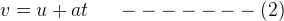This is the velocity and time relation for uniformly accelerated motion. That’s not tough to memorise, though!

Second Equation of Motion: Position – Time Relation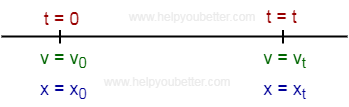Consider a specific object moving along a straight line with constant acceleration ‘a’. Let the object’s velocity be v0, and the position coordinate be x0 at time t = 0. Similarly, after a time interval t, let the object’s velocity be vt and the position coordinate be xt.

Now (xt – x0 ) is called the object’s displacement ‘s’ during this time interval, and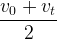is the average velocity.

Then,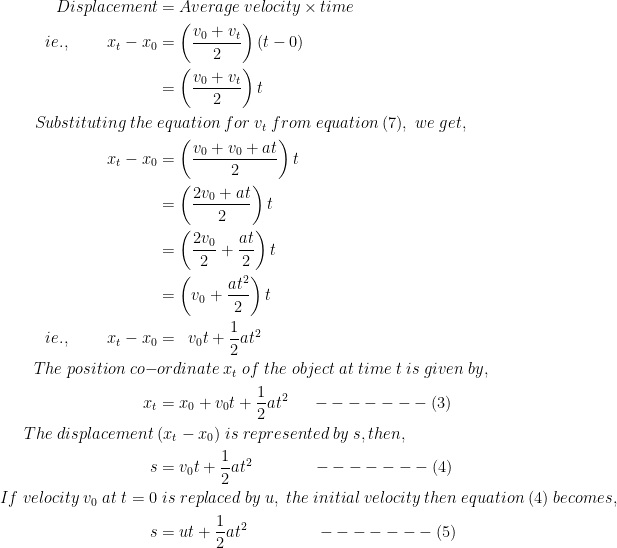AS you can see in equation (3), the first term shows the displacement of our object at t = 0, the second term shows the displacement contribution because of the velocity when acceleration is absent. The third term shows the displacement contributed due to acceleration.

The equation’s third term contains t2, so the position-time graph of the object’s motion will be parabolic in shape, as illustrated in the figure.

Third Equation of Motion: Position – Velocity Relation

Consider a specific object moving along a straight line having constant acceleration ‘a’. Consider v0 as the object’s velocity at time t = 0, and the position coordinate to be x0.

Identically, after an interval of time t, let the object’s velocity be vt while xt to be the position coordinate.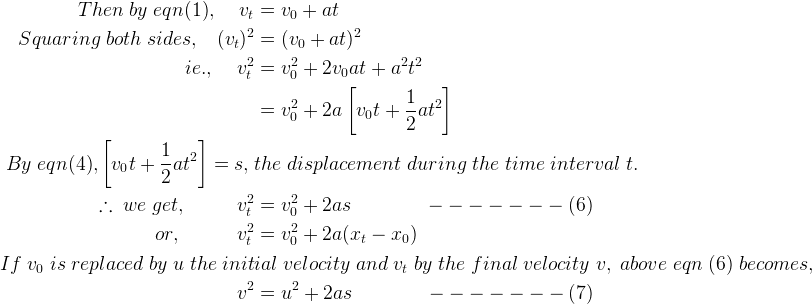Hence, questions 2, 5 and 7 indicate the most general states of equations of motion of a specific object moving along a straight line having constant acceleration.

A vertical motion under gravity

Through his experiment with feathers and coins, Galileo demonstrated that all bodies could reach the Earth simultaneously regardless of their mass. This means that all particles near the Earth's surface feel gravity's force, and this force creates the same acceleration for all particles, regardless of their weights.

The acceleration produced is known as the acceleration caused by gravity (g) and is directed towards the centre of Earth. The acceleration caused by gravity (g) at or close to the Earth's surface is about 9.8 millimetres per second.

When a body can freely fall, the equations of motion are calculated by substituting acceleration(a) in the equation above by the acceleration caused by gravity(g) along with displacement(s) with the magnitude of the fall(h).

So, for downward motion, the equation changes to,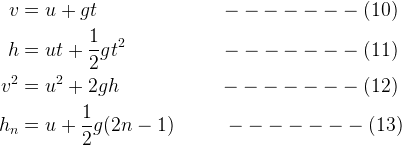Identically, the equations of motion of any particle moving vertically upwards is gained by replacing a by –g.

Hence, for upward motion, the equations are like: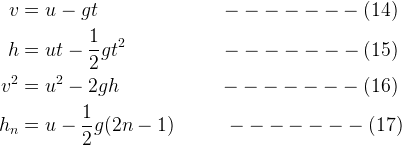Generally, for motion under gravity, the acceleration never changes. It is,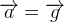. It’s applicable for both downward and upward motion.

Conclusion

Yes, those graphs shown up there can be a bit daunting, but you can practice noting those vital formulae to understand the concept in a quick and consolidated way. Do spend a bit extra time in understanding the Third Equation of Motion.